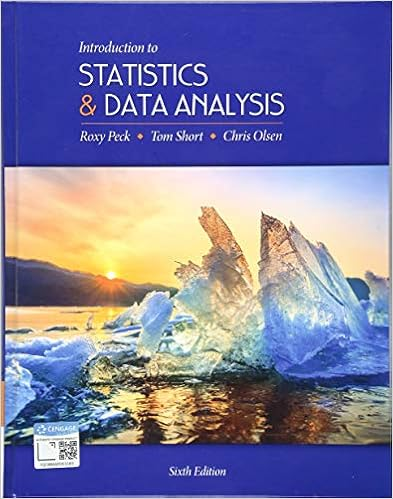# Stats 250 Lecture Notes Chapter 1.pdf - Statistics 250...

• Notes
• 30

This preview shows page 1 - 10 out of 30 pages.

##### We have textbook solutions for you!
The document you are viewing contains questions related to this textbook.The document you are viewing contains questions related to this textbook.
Chapter 7 / Exercise 7.138
Introduction to Statistics and Data Analysis
Olsen/PeckExpert Verified
Statistics 250 Interactive Lecture Notes and Lab Workbook Winter 2019 Used in all lectures and all lab sections of Stats 250 Department of Statistics University of Michigan
##### We have textbook solutions for you!
The document you are viewing contains questions related to this textbook.The document you are viewing contains questions related to this textbook.
Chapter 7 / Exercise 7.138
Introduction to Statistics and Data Analysis
Olsen/PeckExpert Verified
Statistics 250 Interactive Lecture Notes Partial Lecture Notes which you get to co-author as we engage with this content in class.
Topic Page Intro 1 1: Summarizing Data 3 2: Gathering Useful Data 25 3: Probability 37 4: Random Variables 45 5: Learning about a Population Proportion Part 1: Distribution for a Sample Proportion Part 2: Estimating Proportions with Confidence Part 3: Testing about a Population Proportion 69 77 87 6: Learning about the Difference in Population Proportions Part 1: Distribution for a Difference in Sample Proportions Part 2: Confidence Interval for a Difference in Population Proportions Part 3: Testing about a Difference in Population Proportions 99 103 105 7: Learning about a Population Mean Part 1: Distribution for a Sample Mean Part 2: Confidence Interval for a Population Mean Part 3: Testing about a Population Mean 109 117 123 8: Learning about a Population Mean Difference Part 1: Distribution for a Sample Mean Difference Part 2: Confidence Interval for a Population Mean Difference Part 3: Testing about a Population Mean Difference 135 139 143 9: Learning about the Difference in Population Means Part 1: Distribution for a Difference in Sample Means Part 2: Confidence Interval for a Difference in Population Means Part 3: Testing about a Difference in Population Means 147 151 159 10: ANOVA: Analysis of Variance 165 11: Relationships between Quantitative Variables: Regression 179 12: Relationships between Categorical Variables: Chi-Square 201 Brief Index 221
Lecture Notes Page 1 Stats 250 Lecture Notes Introduction Statistics ... the most important science in the whole world : for upon it depends the practical application of every other science and of every art: the one science essential to all political and social administration, all education, all organization based on experience, for it only gives results of our experience." ~ Florence Nightingale, Statistician Definitions: Statistics are numbers measured for some purpose. Statistics is a collection of procedures and principles for gathering data and analyzing information in order to help people make decisions when faced with uncertainty. Course Goal: Learn various tools for using data to gain understanding and make sound decisions about the world around us.
Lecture Notes Page 2
Lecture Notes Page 3 Stats 250 Lecture Notes 1: Summarizing Data “ You must never tell a thing. You must illustrate it. We learn through the eye and not the noggin. " ~ Will Rogers (1879 - 1935) “Simple summaries of data can tell an interesting story and are easier to digest than long lists.” So we will begin by looking at some data. Raw Data Raw data correspond to numbers and category labels that have been collected or measured but have not yet been processed in any way. On the next page is a set of RAW DATA - information about a group of items in this case, individuals. The data set title is DEPRIVED and has information about a sample size of n
•••